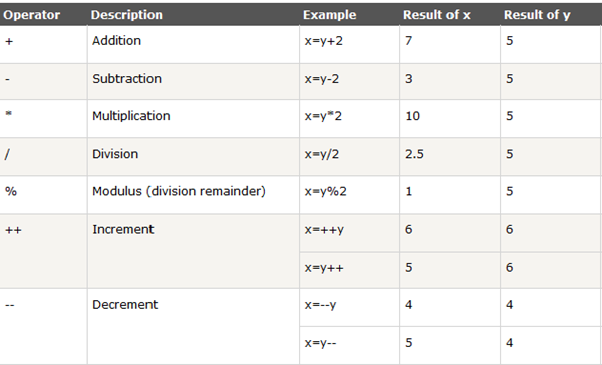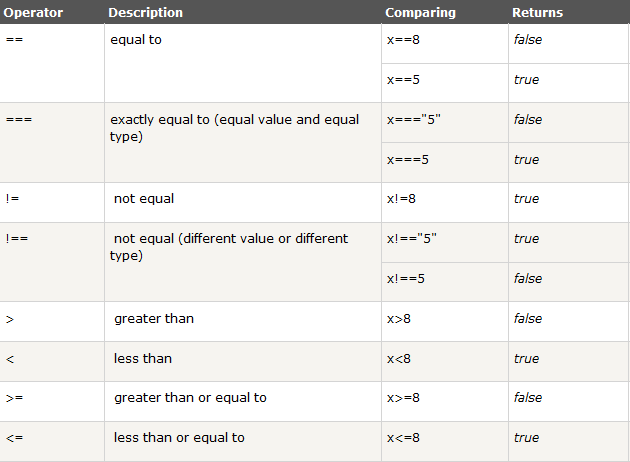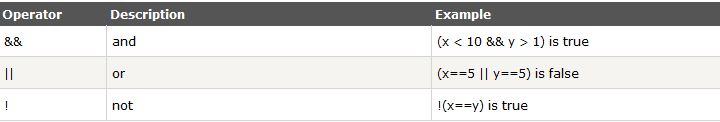## Tuesday, August 13, 2013

### Javascript Variables and Operators

JavaScript Variable Names:
While naming your variables in JavaScript keep following rules in mind.
• You should not use any of the JavaScript reserved keyword as variable name. These keywords are mentioned in the next section. For example, break or boolean variable names are not valid.
• JavaScript variable names should not start with a numeral (0-9). They must begin with a letter or the underscore character. For example, 123test is an invalid variable name but _123test is a valid one.
• JavaScript variable names are case sensitive. For example, Name and name are two different variables.
JavaScript Reserved Words:
The following are reserved words in JavaScript. They cannot be used as JavaScript variables, functions, methods, loop labels, or any object names.
 abstract boolean break byte case catch char class const continue debugger default delete do double else enum export extends false final finally float for function goto if implements import in instanceof int interface long native new null package private protected public return short static super switch synchronized this throw throws transient true try typeof var void volatile while with

Declaring many variables at once
If we want to declare variables, we use the "var" keyword in front of each variable, like this:
var Sam
var Joe
var Bob
var Mary
There is actually a quicker way when it comes to declaring multiple variables, and it looks like this:
var Sam, Joe, Bob, Mary;
Simply use "var" once, followed by the names of the variables, each separated by a semicolon. You can also choose to initialize some/all of the variables upon declaration this way:
var Sam="10yr", Joe="30yr", Bob="15yr", Mary="21yr";

## JavaScript Has Dynamic Types

var x;                              // Now x is undefined
var x = 5;                       // Now x is a Number
var x = "John";           // Now x is a String
var x=true;                  // Now x is a Boolean
var y=false;                 // Now x is a Boolean
var x1=34.00;             // Written with decimals

## JavaScript Arithmetic Operators

Arithmetic operators are used to perform arithmetic between variables and/or values.

Given that y=5, the table below explains the arithmetic operators:## JavaScript Assignment Operators

Assignment operators are used to assign values to JavaScript variables.
Given that x=10 and y=5, the table below explains the assignment operators:

## The + Operator Used on Strings

`var x=5+5;                  //Output: 10`
`var y="5"+5;                //Output: 55  `
`var z="Hello"+5;            // Output: Hello5`
` var a=true+5;              // Output: 6`
` var a=false+5;             // Output: 5`
` var a=""+5;                // Output: 5`
` var a=null+5;              // Output: 5`
`var a="null"+5;             // Output: null5 `
` var x; var z=x+5;          //Output: NaN`

## Comparison Operators

Comparison operators are used in logical statements to determine equality or difference between variables or values.
Given that x=5, the table below explains the comparison operators:## Conditional Operator

### Syntax

variablename=(condition)?value1:value2

### Example

var age=25;

var voteable=(age<18)?"Too young":"Old enough";

## Logical Operators

Logical operators are used to determine the logic between variables or values.
Given that x=6 and y=3, the table below explains the logical operators: